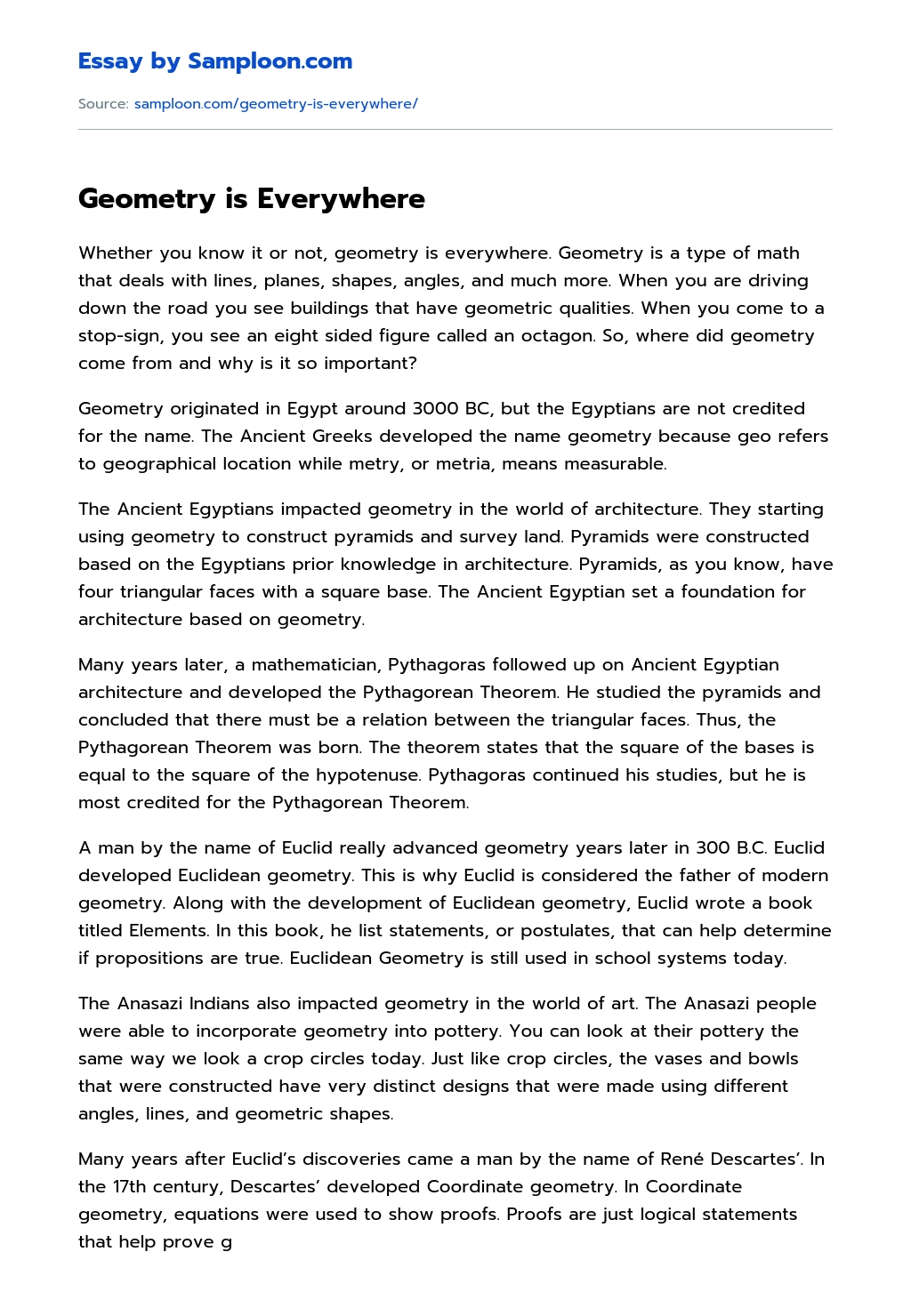# Geometry is Everywhere

Updated October 13, 2020
• Subject
• Category
• Topic
• Pages 4
• Words 798
• Views 72Get help to write your own 100% unique essay

78 writers are online and ready to chat

Whether you know it or not, geometry is everywhere. Geometry is a type of math that deals with lines, planes, shapes, angles, and much more. When you are driving down the road you see buildings that have geometric qualities. When you come to a stop-sign, you see an eight sided figure called an octagon. So, where did geometry come from and why is it so important?

Geometry originated in Egypt around 3000 BC, but the Egyptians are not credited for the name. The Ancient Greeks developed the name geometry because geo refers to geographical location while metry, or metria, means measurable.

The Ancient Egyptians impacted geometry in the world of architecture. They starting using geometry to construct pyramids and survey land. Pyramids were constructed based on the Egyptians prior knowledge in architecture. Pyramids, as you know, have four triangular faces with a square base. The Ancient Egyptian set a foundation for architecture based on geometry.

Many years later, a mathematician, Pythagoras followed up on Ancient Egyptian architecture and developed the Pythagorean Theorem. He studied the pyramids and concluded that there must be a relation between the triangular faces. Thus, the Pythagorean Theorem was born. The theorem states that the square of the bases is equal to the square of the hypotenuse. Pythagoras continued his studies, but he is most credited for the Pythagorean Theorem.

A man by the name of Euclid really advanced geometry years later in 300 B.C. Euclid developed Euclidean geometry. This is why Euclid is considered the father of modern geometry. Along with the development of Euclidean geometry, Euclid wrote a book titled Elements. In this book, he list statements, or postulates, that can help determine if propositions are true. Euclidean Geometry is still used in school systems today.

The Anasazi Indians also impacted geometry in the world of art. The Anasazi people were able to incorporate geometry into pottery. You can look at their pottery the same way we look a crop circles today. Just like crop circles, the vases and bowls that were constructed have very distinct designs that were made using different angles, lines, and geometric shapes.

Many years after Euclid’s discoveries came a man by the name of René Descartes’. In the 17th century, Descartes’ developed Coordinate geometry. In Coordinate geometry, equations were used to show proofs. Proofs are just logical statements that help prove geometric statements. Coordinate geometry was also able to further prove Euclid’s list of postulates. Descartes’ discoveries would later influence famous mathematicians like Sir Isaac Newton.

Because of the discoveries of Pythagoras, Euclid, and Descartes’, geometry is used in the world today. A major part of geometry is used in the workplace. Architects use area and perimeter to determine the size of buildings. Take the St. Louis Arch for example. Geometry played a huge part of the construction process. Every part of the arch had to be angled in such a way that each part would support the other.

Geometry spreads further than the construction of buildings. Many artworks include geometric figures. The canvas can be considered a plane that consist of multiple geometric shapes. These geometric figures help create an overall them throughout the artwork.

In America, geometry is taught to most high school students. Geometry is not a hard concept to grasp, and neither are geometric problems. Say you were told to find the area of square with a length of 5 inches and a width of 9 inches. To solve this problem, you would simply use the formula length times width to get the area which is 45 inches. If you were asked to find the area of a circle with a radius of 3 centimeters, you would have to use a different formula. The area of a circle is equal to pie times the radius squared. So, you would plug 3 in for the radius and end up with the area being equal to 28.27 centimeters.

Throughout my research, I learned that you truly don’t know something until you do actual research on a topic. I was able to learn about the mathematicians that contributed to my B the second nine weeks of school. I learned that Euclid is the man who wrote the first set of postulates that all students love. I also learned that Descartes’ is to credit for my hatred in writing proof statements. To me, it is very interesting how these mathematicians were able to make these discoveries. The earliest mathematicians had no knowledge in the field of geometry, but yet they were able to come up with all sorts of formulas and ways of solving problems.

Overall, geometry is complex in its simplest ways. Geometry is so much more than just shapes. Geometry is a way of life. From the workplace to the classroom, geometry has a lasting impact on our lives.Remember. This is just a sample

You can get your custom paper from our expert writers